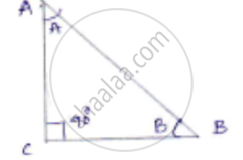Share

# If Angle a and Angle B Are Acute Angles Such that Cos a = Cos B, Then Show that Angle a = Angle B. - CBSE Class 10 - Mathematics

ConceptTrigonometric Ratios of an Acute Angle of a Right-angled Triangle

#### Question

If ∠A and ∠B are acute angles such that cos A = cos B, then show that ∠A = ∠B.

#### Solution

∠A and ∠B are acute angles

Cos A = cos B S.T ∠A = ∠B

Let us consider right angled triangle ACB.We have cos A = "adjacent side"/"Hypotenuse"

= (AC)/(AB)

cos B = (BC)/(AB)

cos A = cos B

(AC)/(AB) = (BC)/(AB)

AC = BC

∠A = ∠B

Is there an error in this question or solution?

#### APPEARS IN

Solution If Angle a and Angle B Are Acute Angles Such that Cos a = Cos B, Then Show that Angle a = Angle B. Concept: Trigonometric Ratios of an Acute Angle of a Right-angled Triangle.
S